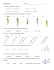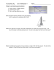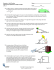# File - HHS Pre

## Transcription

File - HHS Pre
```7.5-7.7 Trig Applications & Area
Pre-AP Geometry
Name ___________________________________
Date: ________ Period: _______
EVALUATE
Find the area of each regular polygon.
1.
2.
3.
10 km
Perimeter = 108 mi
4.
5.
Perimeter = 144 cm
A regular hexagon with a perimeter of 48 yd.
9 cm
6.
A regular pentagon, 6 ft on each side.
Find the area of each figure given below.
7.
8.
10 ft.
35°
25 m
42°
Solve the following Trig Word Problems
1. From a point 115 feet from the base of a redwood tree, the angle of elevation to the top of the tree is 64.3 o. Find
the height of the tree to the nearest foot.
2. From a point 10 feet from the base of a flag pole, the angle of elevation to the
he top of the flag pole is 76.4 o. Find
the height of the flag pole to the nearest foot.
3. DME (Distance Measuring Equipment) is standard avionic equipment on a commercial airplane. This equipment
measures the distance from a plane to a rad
ar station. If the distance from a plane to a radar station is 160 miles
o
and the angle of depression is 33 , find the number of ground miles from a point directly below the plane to the
4. A backpacker notes that from a certain point on leve
levell ground, the angle of elevation to a point
p
at the top of a tree
is 34 o. After walking 50 feet closer to the tree, the backpacker notes the angle of elevation is 54 o. Find the height
of the tree.
5. A submarine traveling 9 mph is descending at an angle o
of depression of 5 o. How many minutes does it take the
submarine to reach the depth of 50 feet?
6. The angle of elevation to the top of the Egyptian pyramid Cheops is 36.4 o, measured from a point 350 feet from
the base of the pyramid. The angle of elevati
elevation of a face of the pyramid is 51.9o. Find the height of Cheops.
7.
During its approach to Earth, the space shuttle's glide angle changes. When the shuttle's altitude is about 14.5 miles, its
horizontal distance to the runway is about 52 miles.
What
at is the glide angle? Round your answer to the nearest tenth.
When the space shuttle is 6 miles from the runway, its glide angle is about 21°. Find the
shuttle's altitude at this point in its descent. Round your answer to the nearest tenth.
8.
The altitude
ude to the base of an isosceles triangle is 17 inches long. The measure of a base
angle is 41°. Find the measure of the base to the nearest inch.
9.
The horizontal part of a step is called the tread. The vertical part is called the riser. The ratio of the riser
Length
ength to the tread length affects the safety of a staircase. Traditionally, builders have used a riser-to-tread
riser
ratio of about 8.25 inches: 9 inches. A newly recommended ratio is 7 inches: 11 inches.
Find the value of x for stairs
irs built using the new riser
10. You are designing a ramp where the horizontal distance is twice as long as the vertical rise. What will be the ramp angle to the
nearest tenth of a degree?
```

### Worksheet - Multi-Step Trigonometry Problem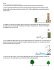### installation of an angle board * * * thermopian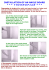### File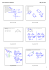### Review12.1-12.6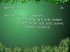### MCR3U - 4.2 - Applications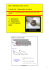### , 660o s = feet = 32.97 feet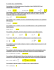### Problem Solving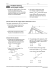### Torque/Cross Product Worksheet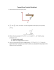### Name______________________________ Worksheet – Solving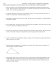### If ABC is obtuse, what are the restrictions on x? ∠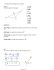### Trig Applications ws day 2 geo 14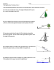### Test 2 Review 1